## Use cross-correlation for speech detection/verification and time delay measurementStarted by 3 years ago14 replieslatest reply 3 years ago285 views
I have been experimenting with cross-correlation function to verify the presence of speech in a recorded file wrt a source file. I tried the following in Matlab:

source = '/home/skrowten-hermit/Programs/male_8k.wav'
silenced = '/home/skrowten-hermit/Programs/male_8k_silenced.wav'
halved = '/home/skrowten-hermit/Programs/male_8k_halved.wav'
attenuated = '/home/skrowten-hermit/Programs/male_8k_attenuated.wav'
plot(y1)
plot(y2)
plot(y3)
plot(y4)

%% autocorrelation function wrt source

% calculate autocorrelation
[Rx1, lags1] = xcorr(y1, 'coeff')
tau1 = lags1/fs1

% plot the signal autocorrelation function
figure(6)
plot(tau1, Rx1, 'r')

%% crosscorrelation function wrt source

% calculate correlation and time axis
[Rx2, lags2] = xcorr(y1, y2, 'none')
tau2 = lags2/fs2
[Rx3, lags3] = xcorr(y1, y3, 'none')
tau3 = lags3/fs3
[Rx4, lags4] = xcorr(y1, y4, 'none')
tau4 = lags4/fs4

% plot the signal correlation function
figure(6)
plot(tau2, Rx2, 'r')
figure(6)
plot(tau3, Rx3, 'r')
figure(6)
plot(tau4, Rx4, 'r')

[pRx1, idx1] = max(Rx1)
[pRx2, idx2] = max(Rx2)
[pRx3, idx3] = max(Rx3)
[pRx4, idx4] = max(Rx4)

fprintf('Peak value of Rx1 is %f at %f', pRx1, lags(idx1))
fprintf('Peak value of Rx2 is %f at %f', pRx2, lags(idx2))
fprintf('Peak value of Rx3 is %f at %f', pRx3, lags(idx3))
fprintf('Peak value of Rx4 is %f at %f', pRx4, lags(idx4))

The following are the waveforms of my input files as generated by Matlab (male_8k.wav is my source from which others are generated or recorded):

### male_8k.wav### male_8k_halved.wav### male_8k_silenced.wav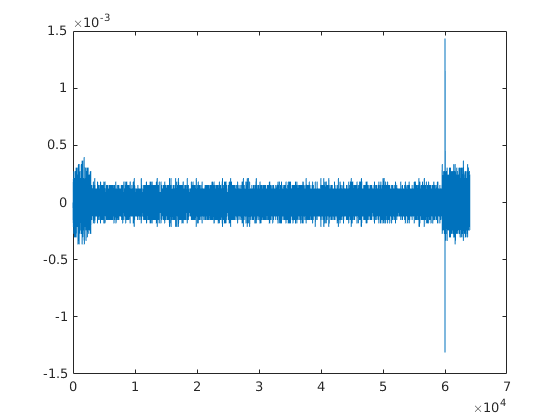### male_8k_attenuated.wavThe auto-correlation (for male_8k.wav) generates the following outputs:The cross-correlation of male_8k.wav with male_8k_halved.wav gives the following: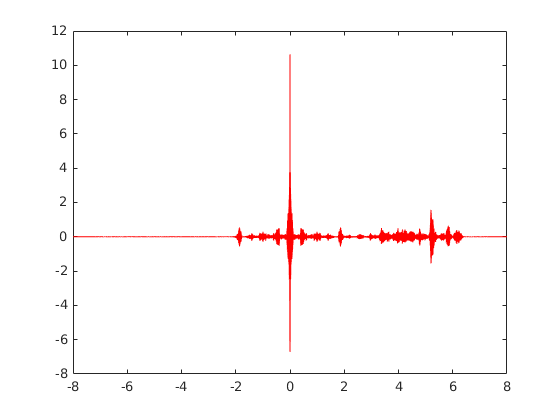The cross-correlation of male_8k.wav with male_8k_silenced.wav gives the following: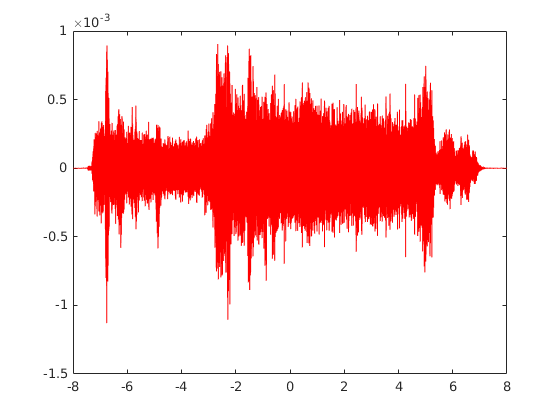The cross-correlation of male_8k.wav with male_8k_attenuated.wav gives the following: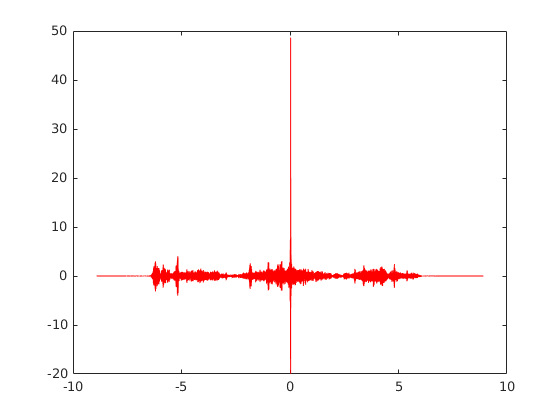The output of peak value and the indices (location) of the peaks are as follows:

Peak value of Rx1 is 1.000000 at 0.000000
Peak value of Rx2 is 10.634055 at 0.000000
Peak value of Rx3 is 0.000905 at -21325.000000
Peak value of Rx4 is 48.637631 at 7516.000000

Since I intend to use the above with recorded files over a network by playing the source file (male_8k.wav) at the transmitter (Tx) end and record at a reciever (Rx) in order to verify if there is some speech detected at Rx and calculate the delay (in ms), I would like to quantify them as success or failure for verification and convert the indices (i.e., the time sample) into a value in ms. I understand that the result (i.e., the peak value) could never be 1 as in ACF, but is it possible to fix a threshold for peak and convert sample number index in such a way that:

1. Normalize the input vectors so that the amplitudes are between -1 and 1.
2. I could distinguish between silence and some speech data (attenuated is fine - just need to check if data samples exist at Rx).
3. I could determine there is a delay of d ms at Rx.

The output values of peaks reading 10.634055 for half the speech data samples and 48.637631 for attenuated speech data samples left me a bit confused. How can I do this effectively/efficiently?

[ - ]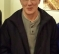Skrowten Hermit-

Your attenuated waveform looks odd -- like it's clipped, not attenuated. Definitely cross-correlating x with an attenuated version of x should always be less than x cross-correlated with itself.

If this is just for network measurement -- using the same input speech segment repeatedly in the presence of different network delay and noise conditions -- your approach may be ok. If you expect the method to hold up if you change the input segment, or test in the presence of other speech, I would advise against relying only on cross-correlation plus a threshold.

As for threshold estimation, that's somewhat a black art. You might try some type of normalization, for example setting the threshold as a percentage of envelope amplitude, or similar.

-Jeff

[ - ]Yeah right? I too thought so. But when I analyse it - it's amplitude is lesser than the original speech file male_8k.wav and it's gain level is also less, -68dB compared to -39dB of the original. Is this okay to be used as an attenuated sample?

I too expected that cross-correlating y1 (original input vector from male_8k.wav) with attenuated y4 would be less than 1 (the peak at 0 as shown in output of auto-correlation of y1Peak value of Rx1 is 1.000000 at 0.000000). Will normalizing the signal vectors help? If it does, how do I do it? Or a normalized cross-correlation is a better approach? Are they different or same?

That's correct. I intend to use the source speech in male_8k.wav to be pumped/played at the Tx over a network (over the air or a VoIP line) and record at an Rx and then just determine if speech exists and the gain wrt the source - i.e., if there is an attenuation or an amplification. So, a basic verification and not a word to word speech detection or speech to text or something like that. There would not be any other background speech as well. Also, would like to calculate the delay as well. So, cross-correlation is the right approach?

So, threshold estimation needs a trial-and-error approach using the amplitude? Could you please elaborate on using percentage of envelope amplitude?

[ - ]Skrowten Hermit-

If it's attenuated in a mathematical sense, then it should have the same shape -- i.e. should look identical except for values on the y-axis. But one you show doesn't.

For normalization methods, the Wikipedia article mentioned by Platybel would be a place to start.

To estimate envelope amplitude of your Rx signal, you could try a running average over, say, 128 samples, and then multiply that by a constant to set an xcorr threshold. This method would update continuously and thus adapt to your Rx input. To test you can bypass the network and insert deliberate attenuation / distortion combinations, and verify it works reliably.

-Jeff

[ - ]So, for the Rx signal I need to choose a window size (like you said, say, 128 samples) and calculate a moving/block average, multiply it by a constant like, say 0.3 (for 30%) to maintain the threshold. Am I right? Can I not directly multiply a constant like 0.3 to the auto-correlation and compare with the cross-correlation? Because I intend to use only cross-correlation for verification.

[ - ]It will help me understand the physics of the problem if you describe a bit about the three (y2,y3,y4)time series that you trying to cross-correlate with the y1.

Crosscorrelation of y1 with y2,y3,y4 will give different peak values, even more than one peak. You could try normalising the cross correlation.  Several normalisation practices (according to different disciplines) are discussed in Wikipedia under 'Cross correlation'.

Then you can set a threshold.

Hope this helps.

[ - ]So, the following are the details of the input signal vectors (all from files of length 8 seconds, mono channel and sampled with a rate of 8 kHz):

1. y1 - the original input signal vector from male_8k.wav. Contains speech data with 2 spoken sentences with a pause between them.
2. y2 - the input signal vector from male_8k_halved.wav. Contains speech data with only the first sentence in the file male_8k.wav.
3. y3 - the input signal vector from male_8k_silenced.wav, a blank file which has no speech, only silence.
4. y4 - the input signal vector from male_8k_attenuated.wav. Contains the speech data from male_8k.wav, recorded on an analog interface and is attenuated/with low gain compared to the original input signal vector from male_8k.wav.
Crosscorrelation of y1 with y2,y3,y4 will give different peak values, even more than one peak.

Can you explain or give pointers on why? With respect to normalization, which would be a better approach:

1. normalizing the input signals say y1 and y2 and apply cross-correlation on the normalized signals or
2. normalizing the cross-correlation

How are they different? Or are they the same?

[ - ]I do not understand why you expect the crosscorrelation of y1 with different time series would give you the same peak value. Crosscorrelation is simply 'lag multiply sum' operation.  Consider p1=[1 2], p2=[3 4], p3=[5 6].

p1 with p2 gives [4 11 6]

p1 with p3 gives [6 17 10]

Matlab xcorr has an option 'normalized'.  xcorr(p1,p2) & xcorr(p1,p3) give the above results. The normalized option always has the peak value =< 1.

xcorr(p1,p2,'normalized') gives [0.3578 0.9839 0.5637]

[ - ]Thanks for the pointer on normalization.

Sorry, I meant on the number of correlation coefficients in the correlation vector. I understand that the peaks would be different. Matlab's xcorr function has a maxLag parameter that computes cross-correlation from -maxLag to maxLag. Doesn't that correspond to a limit? I understand that the length of auto-correlation is always 2N+1 (-N to N) for an input of N samples. When it comes to cross-correlation for x and y with samples N and M respectively, my understanding was that I should always zero-pad the lesser among N and M and compute the cross-correlation. But the maxLag has made me a bit confused. Should I just pass the greater among N and M as maxLag?

[ - ]Let us consider a short vector A=[0 1 2 3 0] and a vector B (see matlab script below).  I have deliberately chosen the lengths of A (5) & B (21) as odd.  But one can work out for even numbers easily.

We crosscorrelate C=AxB, the process is simply (overlap> shift> multiply> add).  So we can compute manually:

Cmanual(1) = A(5)xB(1) (the x here is multiplication).

Cmanual(2) = A(4)xB(1)+A(5)xB(2)

......

Cmanual(24) = A(1)xB(20)+A(2)xB(21)

Cmanual(25) = A(1)xB(21)

The length of Cmanual is M+N-1=21+5-1=25

But matlab xcorr gives M+M-1, 41 points.  Matlab zeropads the shorter vector to make both the same length and carry out the (shift multiply add) efficiently in the frequency domain, with the help of FFT.  But it is not necessary to understand the inner workings of xcorr to use it.

If you carried out the cross correlation manually, you will get M+N-1 points, not M+M-1.

In the matlab code below, we have Cxcorr as the output of xcorr.

If you want to get the manual crosscorrelation, as you would have done it by hand, just truncate to  M+N-1  points, as in Cmanual

The purpose of crosscorrelation of A with B is to find if there is a region similar to A in B.  But that question cannot be answered unless A and B are in full overlap.  So of the M+N-1 points in Cmanual, we have to select only those points for which A & B fully overlap.  That is in Cmaxlag.

In xcorr() the maxlag parameter can be set to any +/- lag value.  I normally set it to the lag upto which we have complete overlap of A and B.

To repeat Cmaxlag is a subset of Cmanual which is a subset of Cxcorr.

I hope I have clarified your doubts.  If not, don't hesitate to write

clear all; close all;
N=5,M=21,
%time series A
%x=[1:19];
x1=[fix((-M-N)/2)+1:fix((M+N-1)/2)];
x2=[-fix((M-N)/2):fix(M/2-N/2)];
A=zeros(1,5);
A(2:4)=[1 2 3];
B=zeros(1,M);
B(7:11)=A.^(0.5);
[Cxcorr,lag]=xcorr(A,B)
Cmanual=Cxcorr(1:N+M-1)
Cmaxlag=Cxcorr(N:M);

figure
subplot(5,1,1)
plot(A,'r')
title('A')
xlabel('index')
subplot(5,1,2)
plot(B,'r')
title('B')
xlabel('index')
subplot(5,1,3)
plot(lag,Cxcorr,'r')
xlabel('lag')
title('Cxcorr')
subplot(5,1,4)
plot(x1,Cmanual,'r')
title('Cmanual')
xlabel('lag')
subplot(5,1,5)
plot(x2,Cmaxlag,'r')
title('Cmaxlag')
xlabel('lag')[ - ]Thanks a lot. This helped. Exactly what I wanted to understand regarding the maxLag parameter of xcorr.

[ - ]The 1957 Plymouth Belvedere had a self-seeking radio, with buttons labelled 'Speech' and 'Music' It would accurately find radio stations with either type of program running, without a single semiconductor or line of software in sight.

Just thought you'd like to know :)

[ - ]Very interesting - all at analog level?

I'm sensing that this could be a hint for me?

[ - ]All with thermionic valves. I suspect there were a few well-designed filters in there somewhere.

It also had a push-button automatic transmission, but we'll ignore that...

[ - ]